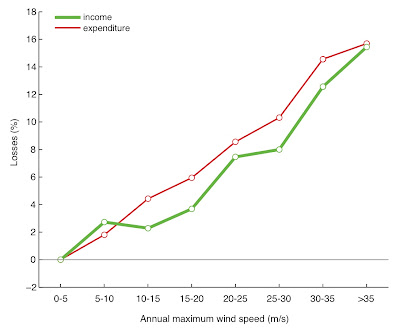## 1.23.2013

### Binning a continuous independent variable for flexible nonparametric models [in Stata]

Sometimes we want to a flexible statistical model to allow for non-linearities (or to test if an observed relationship is actually linear). It's easy to run a model containing a high-degree polynomial (or something similar), but these can become complicated to interpret if the model contains many controls, such as location-specific fixed effects. Fully non-parametric models can be nice, but they require partialling out the data and standard errors can be come awkward if the sample is large or something sophisticated (like accounting for spatial correlation) is required.

An alternative that is easy to interpret, and handles large samples and complex standard errors well, is to convert the independent variable into discrete bins and to regress the outcome variable on dummy variables that represent each bin.

For example, in a paper with Jesse we take the typhoon exposure of Filipino households (a continuous variable) and make dummy variables for each 5 m/s bin of exposure. So there is a 10_to_15_ms dummy variable that is zero for all households except for those households whose exposure was between 10 and 15 m/s, and there is a different dummy for exposure between 15 and 20 m/s, etc.  When we regress our outcomes on all these dummy variables (and controls) at the same time, we recover their respective coefficients -- which together describe the nonlinear response of the outcome. In this case, the response turned out to be basically linear:The effect of typhoon exposure on Filipino households finances. From Anttila-Hughes & Hsiang (2011)

This approach coarsens the data somewhat, so there is some efficiency loss and we should be wary of Type 2 error if we compare bins to one another. But as an approach to determine the functional form of a model, this is a great approach so long as you have enough data.

I found myself rewriting Stata code to bin variables like this in many different contexts, so I wrote bin_parameter.ado to do it for me quickly.  Running these models can now be done in two lines of code (one of which is the regression command). bin_parameter allows you to specify a bin width, a top bin, a bottom bin and a dropped bin (for your comparison group). It spits out a bunch of dummy variables that represent all the bins which cover the range of the specified variable. It also has options for naming the dummy variables so you can use the wildcard notation in regression commands. Here's a short example of how it can be used:

set obs 1000
gen x = 10*runiform()-5
gen y = x^2
bin_parameter x, s(1) t(4) b(-4) drop(-1.6) pref(x_dummy)
reg y x_dummy*

Help file below the fold.

/* ====================================================

S. HSIANG
SHSIANG@PRINCETON.EDU
9/2012

-------------------------------------------------------

SYNTAX

bin_parameter X [if], Size(integer) Top_bin_lower_bound(integer) Bottom_bin_upper_bound(integer) DROPped_bin(real) [PREF(string) NONAME NODROP]

-------------------------------------------------------

BIN_PARAMETER takes variable X and generates a sequence of dummy variables for binned values of X. The edges of the bins are determined by supplied arguments.

The new dummy variables have a common prefix that may contain the variable name as well as the range of each bin. The prefix and inclusion of the variable name are options that can be changed.

If the edges of the bin land on values of X that are negative, the new dummy vars are labeled with an "M" instead of a minus sign, since the minus sign is not allowed in variable names.

A specified bin is dropped as the comparison group, unless the option NODROP is specified.

-------------------------------------------------------

Required arguments:

Size - width of bins (default = 1)

Top_bin_lower_bound - lower cutoff for maximum bin, all values above this number are binned

Bottom_bin_upper_bound - top cutoff for minimum bin, all values below this number are binned

DROPped_bin - value of X that denotes which bin is dropped. The bin that contains this value will be dropped. Example: if DROP(1) is specified and there is a bin for values of 0 < x < 3, than this bin will be dropped.

Options:

PREF() - a string that changes the prefix on the variable names for the generated dummy vars (default prefix is "_bin")

NONAME - if specified, the new dummy vars will not have the variable name for X used after the prefix in their names

NODROP - prevents any bin from being dropped. If NODROP is specified, then no bin will be dropped and all coefficients in later estimates will represent comparisons with zero, rather than the dropped bin. In these cases, DROP() must still be specified with a real argument, however the value of the argument is irrelevant.

-------------------------------------------------------

The functions PLOT_RESPONSE and PARM_BIN_CENTER are designed to be used along with BIN_PARAMETER, following regression estimations.

-------------------------------------------------------

CITATION IF USED FOR PUBLICATION:

Hsiang, Lobell, Roberts and Schlenker, 2012: "Climate and the Location of Crops"

-------------------------------------------------------

Example:

bin_parameter X, size(2) t(20) b(4) drop(5) pref(_dummy_var)

======================================================*/